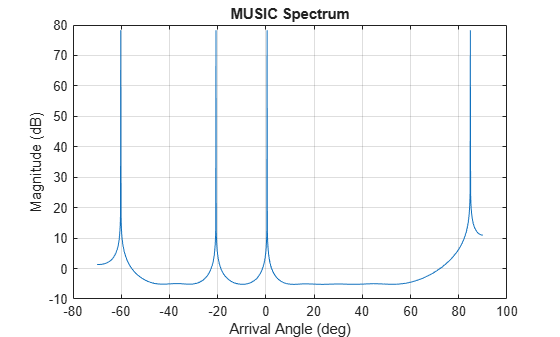# musicdoa

Estimate arrival directions of signals using MUSIC

## Syntax

``doas = musicdoa(covmat,nsig)``
``````[doas,spec,specang] = musicdoa(covmat,nsig)``````
``[___] = musicdoa(covmat,nsig,___,'ScanAngles',scanangle)``
``[___] = musicdoa(covmat,nsig,___,'ElementSpacing',dist)``

## Description

example

````doas = musicdoa(covmat,nsig)` uses the MUSIC algorithm to estimate the directions of arrival, `doas`, of `nsig` plane waves received on a uniform linear array (ULA). The argument `covmat` is a positive-definite Hermitian matrix representing the sensor covariance matrix. Detected sources appear as peaks in the spatial spectrum. The argument `nsig` is the number of arriving signals. Sensor elements are spaced one-half wavelength apart in units of wavelengths. The function forces exact conjugate symmetry of `covmat` by averaging the covariance matrix with its conjugate transpose.```

example

``````[doas,spec,specang] = musicdoa(covmat,nsig)``` also returns the spatial spectrum, `spec`, and the `nsig` angles of the spectrum peaks, `specang`.```

example

````[___] = musicdoa(covmat,nsig,___,'ScanAngles',scanangle)` specifies the grid of broadside angles to search for spectrum peaks.```

example

````[___] = musicdoa(covmat,nsig,___,'ElementSpacing',dist)` specifies the spacing between array elements.```

## Examples

collapse all

Calculate the directions of arrival of 3 uncorrelated signals arriving at an 11-element ULA with half-wavelength spacing. Assume the signals are coming from the broadside angles of 0°, –12°, and 85°. The noise at each element is Gaussian white noise and is uncorrelated between elements. The SNR is 5 dB.

Specify the number of ULA elements and the element spacing (in wavelengths).

```nelem = 11; d = 0.5; snr = 5.0; elementPos = (0:nelem-1)*d;```

Specify the number of signals and their broadside arrival angles.

```nsig = 3; angles = [0.0 -12.0 85.0];```

Create the sensor covariance matrix.

`covmat = sensorcov(elementPos,angles,db2pow(-snr));`

`doas = musicdoa(covmat,nsig)`
```doas = 1×3 0 85 -12 ```

The estimated angles match the specified angles.

Calculate the directions of arrival of 3 uncorrelated signals arriving at an 11-element ULA with half-wavelength spacing. Assume the signals are coming from the broadside angles of 0°, –12°, and 85°. The noise at each element is Gaussian white noise and is uncorrelated between elements. The SNR is 2 dB.

Specify the number of ULA elements and the element spacing (in wavelengths).

```nelem = 11; d = 0.5; snr = 2.0; elementPos = (0:nelem-1)*d;```

Specify the number of signals and their broadside arrival angles.

```nsig = 3; angles = [0.0 -12.0 85.0];```

Create the sensor covariance matrix.

`covmat = sensorcov(elementPos,angles,db2pow(-snr));`

Compute the MUSIC spectrum and estimate the broadside arrival angles.

`[doas,spec,specang] = musicdoa(covmat,nsig);`

Plot the MUSIC spectrum.

```plot(specang,10*log10(spec)) xlabel('Arrival Angle (deg)') ylabel('Magnitude (dB)') title('MUSIC Spectrum') grid```The estimated angles match the specified angles.

Calculate the directions of arrival of 4 uncorrelated signals arriving at an 11-element ULA. The element spacing is 0.5 wavelengths. Assume the signals are coming from the broadside angles of –60.2°, –20.7°, 0.5°, and 84.8°. The noise at each element is Gaussian white noise and is uncorrelated between elements. The SNR is 0 dB.

Specify the number of ULA elements and the element spacing (in wavelengths).

```nelem = 11; d = 0.5; snr = 5.0; elementPos = (0:nelem-1)*d;```

Specify the number of signals and their broadside arrival angles.

```nsig = 4; angles = [-60.2 -20.7 0.5 84.8];```

Create the sensor covariance matrix.

`covmat = sensorcov(elementPos,angles,db2pow(-snr));`

Compute the MUSIC spectrum and estimate the broadside arrival angles in the range from -70° to 90° in 0.1° increments.

`[doas,spec,specang] = musicdoa(covmat,nsig,'ScanAngles',[-70:.1:90]);`

Plot the MUSIC spectrum.

```plot(specang,10*log10(spec)) xlabel('Arrival Angle (deg)') ylabel('Magnitude (dB)') title('MUSIC Spectrum') grid````disp(doas)`
``` 84.8000 -60.2000 0.5000 -20.7000 ```

The estimated angles match the specified angles.

Calculate the directions of arrival of 4 uncorrelated signals arriving at an 11-element ULA. The element spacing is 0.4 wavelengths spacing. Assume the signals are coming from the broadside angles of –60°, –20°, 0°, and 85°. The noise at each element is Gaussian white noise and is uncorrelated between elements. The SNR is 0 dB.

Specify the number of ULA elements and the element spacing (in wavelengths).

```nelem = 11; d = 0.4; snr = 0.0; elementPos = (0:nelem-1)*d;```

Specify the number of signals and their broadside arrival angles.

```nsig = 4; angles = [-60.0 -20.0 0.0 85.0];```

Create the sensor covariance matrix.

`covmat = sensorcov(elementPos,angles,db2pow(-snr));`

Compute the MUSIC spectrum and estimate the broadside arrival angles.

`[doas,spec,specang] = musicdoa(covmat,nsig,'ElementSpacing',d);`

Plot the MUSIC spectrum.

```plot(specang,10*log10(spec)) xlabel('Arrival Angle (deg)') ylabel('Magnitude (dB)') title('MUSIC Spectrum') grid```The estimated angles match the specified angles.

## Input Arguments

collapse all

Sensor covariance matrix, specified as a complex-valued, positive-definite M-by-M matrix. The quantity M is the number of elements in the ULA array. The function forces Hermiticity property by averaging the matrix and its conjugate transpose.

Data Types: `double`
Complex Number Support: Yes

Number of arriving signals, specified as a positive integer. The number of signals must be smaller than the number of elements in the ULA array.

Example: `2`

Data Types: `double`

Broadside search angles, specified as a real-valued vector. Angles must lie in the range (–90°,90°) and must be in increasing order.

Example: `[-40:0.5:50]`

Data Types: `double`

Distance between array elements, specified as a real-valued positive scalar.

Example: `0.45`

Data Types: `double`

## Output Arguments

collapse all

Directions of arrival angle, returned as a real-valued 1-by-D vector, where D is the number of arriving signals specified in `nsig`. Angle units are in degrees. Angle values lie in the range specified by `scanangle`.

Spatial spectrum, returned as a positive real-valued vector. The dimension of `spec` equals the dimension of `scanangle`.

Broadside angle of spectrum, returned as a real-valued vector. The dimension of `specang` equals the dimension of `scanangle`.

## Version History

Introduced in R2016b